# Build your First Deep Learning Neural Network Model using Keras in Python

Hello,

Welcome to my first blog of learning. I have chosen my today’s topic as Neural Network because it is most the fascinating learning model in the world of data science and starters in Data Science think that Neural Network is difficult and its understanding requires knowledge of neurons, perceptron and blaahhhh…There is nothing like that, I have been working on Neural Network for quite a few month now and realized that it is so easy. I am just covering Artificial Neural Network in this tutorial and will come up with Convolutional Neural Network very soon. Follow below step to build your NN model.

Data and Business Problem:

Our basic aim is to predict customer churn for a certain bank i.e. which customer is going to leave this bank service. Dataset is small(for learning purpose) and contains 10000 rows with 14 columns. I am not explaining data in detail as dataset is self explanatory. You can download data from my drive.

Prerequisite: I am using Spyder by Anaconda, so you also need to install same (from here). You need to install Tensorflow, Theano and Keras libraries in spyder, follow the steps in my other blog over here. You must be thinking that we are performing analysis using Keras then why do we need Tensorflow and Theano? The fact of the matter is Keras is built on top of Tensorflow and Theano so this 2 insane library will be running in back-end whenever you run the program in Keras.

Deep understanding of NN(you can skip this if you don’t want to learn in depth)

Step 1: Importing data. Pandas DataFrame gives massive functionality to work on data thus, here we are using pandas to import data.

`# Importing the libraries    import numpy as np    import matplotlib.pyplot as plt    import pandas as pd# Importing the dataset    dataset = pd.read_csv('Churn_Modelling.csv')`

Step 2: Create matrix of features and matrix of target variable. In this case we are excluding column 1 & 2 as those are ‘row_number’ and ‘customerid’ which are not useful in our analysis. Column 14, ‘Exited’ is our Target Variable

(Note: Python Indexing start with 0)

`X = dataset.iloc[:, 3:13].valuesy = dataset.iloc[:, 13].values`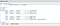Initial Look of data

Step 3: Let’s make analysis simpler by encoding string variables. Country has string labels such as “France, Spain, Germany” while Gender has “Male, Female”. We have to encode this strings into numeric and we can simply do it using pandas but here I am introducing new library called ‘ScikitLearn which is strongest machine learning library in python. We will use LabelEncoder. As the name suggests, whenever we pass a variable to this function, this function will automatically encode different labels in that column with values between 0 to n_classes-1.

`from sklearn.preprocessing import LabelEncoder, OneHotEncoderlabelencoder_X_1 = LabelEncoder()X[:, 1] = labelencoder_X_1.fit_transform(X[:, 1])labelencoder_X_2 = LabelEncoder()X[:, 2] = labelencoder_X_2.fit_transform(X[:, 2])`

Now you can see that Country names are replaced by 0,1 and 2 while male and female are replaced by 0 and 1.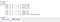Label Encoded Data

Label encoding has introduced new problem in our data. LabelEncoder has replaced France with 0, Germany 1 and Spain 2 but Germany is not higher than France and France is not smaller than Spain so we need to create a dummy variable for Country. Dummy variable is difficult concept if you read in depth but don’t take tension, I have found this simple resource which will help you in understanding. We don’t need to do same for Gender Variable as it is binary.

Step 4: How to create dummy variable in python? We will use the same ScikitLearn library but this time we will use another function called as OneHotEncoder, yeah it is seriously hot. We just need to pass the column number and whoosh your dummy variable is created.

`onehotencoder = OneHotEncoder(categorical_features = )X = onehotencoder.fit_transform(X).toarray()X = X[:, 1:]`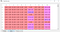Now first 2 columns represent Country and 4th column represent Gender

In Machine Learning, we always divide our data into training and testing part meaning that we train our model on training data and then we check the accuracy of a model on testing data. Testing your model on testing data will only help you evaluate the efficiency of model.

Step 5: We will make use of ScikitLearn’s train_test_split function to divide our data. Roughly people keep 80:20, 75:25, 60:40 as their train test split ratio. Here we are keeping it as 80:20.

`# Splitting the dataset into the Training set and Test setfrom sklearn.model_selection import train_test_splitX_train, X_test, y_train, y_test = train_test_split(X, y, test_size = 0.2)`

I know you are tired of data preprocessing but I promise this is the last step. If you carefully observe data, you will find that data is not scaled properly. Some variable has value in thousands while some have value is tens or ones. We don’t want any of our variable to dominate on other so let’s go and scale data.

Step 6: StandardScaleris available in ScikitLearn. In the following code we are fitting and transforming StandardScaler method on train data. We have to standardize our scaling so we will use the same fitted method to transform/scale test data.

`# Feature Scalingfrom sklearn.preprocessing import StandardScalersc = StandardScaler()X_train = sc.fit_transform(X_train)X_test = sc.transform(X_test)`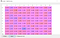Now have a look on data, it is scaled properly

Finally we are done with our data pre-processing now we will start with our model.

I hope you have gone through my another blog for the understanding of concepts of Neural Network. Here we will directly jump on building Neural Network as this blog focuses more on programming.

Let’s begin building long awaited excited Neural Network.

Step 7: Importing required Modules. We need Sequential module for initializing NN and dense module to add Hidden Layers.

`# Importing the Keras libraries and packagesimport kerasfrom keras.models import Sequentialfrom keras.layers import Dense`

Step 8: I am giving the name of model as Classifier as our business problem is the classification of customer churn. In the last step, I mentioned that we will use Sequential module for initialization so here it is:

`#Initializing Neural Networkclassifier = Sequential()`

Step 9: Adding multiple hidden layer will take bit effort. We will add hidden layers one by one using dense function. In the below code you will see a lot of arguments. Don’t worry I will explain them all.

Our first parameter is output_dim. It is simply the number of nodes you want to add to this layer. init is the initialization of Stochastic Gradient Decent. In Neural Network we need to assign weights to each mode which is nothing but importance of that node. At the time of initialization, weights should be close to 0 and we will randomly initialize weights using uniform function. input_dim parameter is needed only for first layer as model doesn’t know the number of our input variables. Remember in our case, the total number of input variables are 11. In the second layer model automatically knows the number of input variable from the first hidden layer.

Activation Function: Very important to understand. Neuron applies activation function to weighted sum(summation of Wi * Xi where w is weight, X is input variable and i is suffix of W and X). The closer the activation function value to 1 the more activated is the neuron and more the neuron passes the signal. Which activation function should be used is critical task. Here we are using rectifier(relu) function in our hidden layer and Sigmoid function in our output layer as we want binary result from output layer but if the number of categories in output layer is more than 2 then use SoftMax function.

Note: Explaining which output function to use is beyond the scope of this blog, if you have any questions then please leave the comment and I will get back to you asap.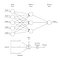Neural Network Diagram
`# Adding the input layer and the first hidden layerclassifier.add(Dense(output_dim = 6, init = 'uniform', activation = 'relu', input_dim = 11))# Adding the second hidden layerclassifier.add(Dense(output_dim = 6, init = 'uniform', activation = 'relu'))# Adding the output layerclassifier.add(Dense(output_dim = 1, init = 'uniform', activation = 'sigmoid'))`

Step 10: Till now we have added multiple layers to out classifier now let’s compile them which can be done using compile method. Arguments added in final compilation will control whole neural network so be careful on this step. I will briefly explain arguments.

First argument is Optimizer, this is nothing but the algorithm you wanna use to find optimal set of weights(Note that in step 9 we just initialized weights now we are applying some sort of algorithm which will optimize weights in turn making out neural network more powerful. This algorithm is Stochastic Gradient descent(SGD). Among several types of SGD algorithm the one which we will use is ‘Adam’. If you go in deeper detail of SGD, you will find that SGD depends on loss thus our second parameter is loss. Since out dependent variable is binary, we will have to use logarithmic loss function called ‘binary_crossentropy’, if our dependent variable has more than 2 categories in output then use ‘categorical_crossentropy’. We want to improve performance of our neural network based on accuracy so add metrics as accuracy

`# Compiling Neural Networkclassifier.compile(optimizer = 'adam', loss = 'binary_crossentropy', metrics = ['accuracy'])`

Congratulations, you have build your first Deep Learning Neural Network model.

Step 11: We will now train our model on training data but still one thing is remaining. We use fit method to the fit our model In previous some steps I said that we will be optimizing our weights to improve model efficiency so when are we updating out weights? Batch size is used to specify the number of observation after which you want to update weight. Epoch is nothing but the total number of iterations. Choosing the value of batch size and epoch is trial and error there is no specific rule for that.

`# Fitting our model classifier.fit(X_train, y_train, batch_size = 10, nb_epoch = 100)`

Step 12: Predicting the test set result. The prediction result will give you probability of the customer leaving the company. We will convert that probability into binary 0 and 1.

`# Predicting the Test set resultsy_pred = classifier.predict(X_test)y_pred = (y_pred > 0.5)`

Step 13: This is the final step where we are evaluating our model performance. We already have original results and thus we can build confusion matrix to check the accuracy of model.

`# Creating the Confusion Matrixfrom sklearn.metrics import confusion_matrixcm = confusion_matrix(y_test, y_pred)`

Result: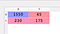Confusion Matrix

So the Accuracy of our model can be calculated as:

Accuracy= 1550+175/2000=0.8625

Awesome, we achieved 86.25% accuracy which is quite good.

It was amazing sharing Neural Network with you guys. I hope this article helped you. Follow me for updates of Machine Learning Blogs and please leave the comment if you want to suggest something.

Best of Luck!

Thanks,

Pushkar Mandot

Source: Udemy Machine Learning Course by kirill eremenko

Machine and Deep Learning Enthusiast, Data Scientist